@Pigmon 2017-04-03T16:22:20.000000Z 字数 8959 阅读 199

# 摄像机标定整理

CV

## 针孔相机模型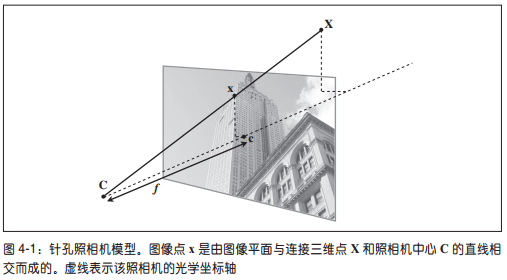$X$：三维点
$x$：图像点
$C$：相机中心
$c$：光心（主点）
$f$：焦距

### 三维点$X$到图像点$x$的投影计算

$\lambda x=PX$ (4.1)
$X$：三维点
$x$：图像点
$\lambda$：三维点$X$的逆深度
$P$：相机矩阵（投影矩阵）,3x4矩阵

### 相机矩阵$P$的计算

$P=K[R|t]$
$K$：标定矩阵，描述照相机的投影性质
$R$：旋转矩阵 3x3
$t$：平移向量 3x1

### 标定矩阵$K$

$K={\begin{bmatrix} \alpha f & s & c_x \\ 0 & f & c_y \\ 0 & 0 & 1 \end{bmatrix}}$
$\alpha$：纵横比例参数，在像素非正方形时使用，所以一般情况都是$1$
$s$：倾斜参数，表示像素数组在传感器上偏斜，所以一般情况都是$0$

$K={\begin{bmatrix} f & 0 & c_x \\ 0 & f & c_y \\ 0 & 0 & 1 \end{bmatrix}}$
$c_x$$c_y$：光心的$x$$y$坐标，通常情况下是图像的中心，可以由图像尺寸计算出来。

### 计算照相机中心$C$

$C$是一个三维点，满足$PC=0$

$\therefore C=-R^Tt$

## 以平面和标记物进行姿态估计

• 拍摄一个矩形参照物正面的照片1
• 拍摄任意角度矩形参照物的照片2
• 提取两个图形的SIFT特征，进行图像配准
• 用RANSAC估计出两幅图像变换的单应性矩阵$H$
• 定义照片1的三维坐标系，通过单应性矩阵映射出照片2对应的坐标系
• 令照片1中标记物平面为$Z=0$，设照片1的相机矩阵$P1={\begin{bmatrix} 1 & 0 & 0 & 0\\ 0 & 1 & 0 & 0 \\ 0 & 0 & 1 & -1\end{bmatrix}}$，计算过程待补充。
• 根据公式$P2=HP1$计算照片2的相机矩阵$P2$

# 三维重建（多视图几何）

## 外极几何 (Epipolar Geometry)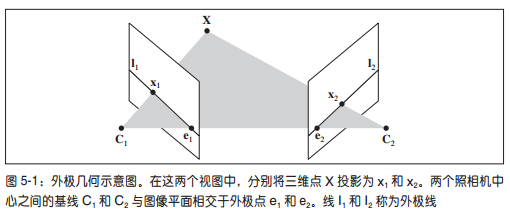$X$：三维点
$C_1$：图像1的摄像机中心
$C_2$：图像2的摄像机中心
$x_1$：图像1的图像点
$x_2$：图像2的图像点
$l_1$：图像1的外极线
$l_2$：图像2的外极线
$e_1$：图像1的外极点
$e_2$：图像1的外极点

### 基础矩阵$F$ （Fundamental Metrix, Essential Matrix）

$P1=K1[I|0]$
$P2=K2[R|t]$

$x_2^TFx_1=0$ (外极约束条件公式，5.1)

$F=K_2^{-T}S_tRK_1^{-1}$ (行列式为0，秩小于等于2)

$S_t={\begin{bmatrix} 0 & -t_3 & t_2 \\ t_3 & 0 & -t_1 \\ -t_2 & t_1 & 0 \end{bmatrix}}$ (5.2)

## 八点法(8-points Algorithm)

### 基本八点法的步骤

$m_2^TFm_1=0$

$F={\begin{bmatrix} f_1 & f_2 & f_3 \\ f_4 & f_5 & f_6 \\ f_7 & f_8 & f_9 \end{bmatrix}}$

$x_1x_2f_1+x_2y_1f_2+x_2f_3+x_1y_2f_4+y_1y_2f_5+y_2f_6+x_1f_7+y_1f_8+f_9=0$

$f$$F$ 的列向量模式，即：
$f=[f_1, f_2, f_3, f_4, f_5, f_6, f_7, f_8, f_9]^T$

$\tilde{m}=[x_1x_2,x_2y_1,x_2,x_1y_2,y_1y_2,y_2,x_1,y_1,1]^T$

$\tilde{m}f=0$----------------------------------------(式1)

$Af=0$----------------------------------------(式2)

$A$是一个$n\times9$矩阵，根据基础矩阵的定义，所有的解向量$f$之间只相差一个常数因子(Why?)。为避免多余解，附加约束条件$||f||=1$，则$f$就是$A^TA$最小特征值对应的特征向量。（待补充）

$A$$SVD$(奇异值分解)为$A=UDV^T$，其中$V=[v_1,...v_9]^T$，则$f=v_9$，即求出了$F$。（待补充）

## 零空间

$Null(A)={v\in V:Av=0}$

## 凸包（Convex Hull）

$X$的凸包可以用X内所有点$(X1，...Xn)$的线性组合来构造。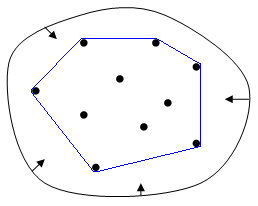## 三角剖分（Triangulation）

1.除了端点，平面图中的边不包含点集中的任何点。
2.没有相交边。
3.平面图中所有的面都是三角面，且所有三角面的合集是散点集$V$的凸包。

## 由三维点计算照相机矩阵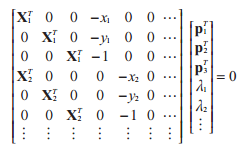$Mv=0$

## 由基础矩阵计算照相机矩阵

### 未标定的情况——投影重建

$P2=[SeF|e]$

$S_t={\begin{bmatrix} 0 & -t_3 & t_2 \\ t_3 & 0 & -t_1 \\ -t_2 & t_1 & 0 \end{bmatrix}}$ (5.2)

### 已标定的情况——度量重建

$x_K = K^{-1}K[R|t]X = [R|t]X$

$x_{K_2}^TFx_{K_1} = 0$

## 多视图重建

(1) 检测特征点，然后在两幅图像间匹配；
(2) 由匹配计算基础矩阵；
(3) 由基础矩阵计算照相机矩阵；
(4) 三角剖分这些三维点。

## 立体视觉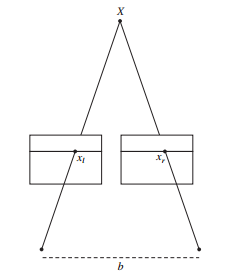$Z=\frac{fb}{x_l-x_r}$

## 图像间的匹配点检测

### 兴趣点描述子

Harris 角点的描述子通常由2部分：

• 角点为中心的像素块（nxn矩形区域）
• 归一化的互相关矩阵（用于比较）

$I_1和I_2$的相关性数值是通过归一化相关矩阵给出的。

### 相关矩阵

$c(I_1, I_2)=\Sigma_x f(I_1(x), I_2(x))$

### 归一化相关矩阵

$ncc(I_1,I_2)=\frac{1}{n-1} \Sigma_x [\frac{(I_1(x)-\mu_1)}{\sigma_1} \frac{(I_2(x)-\mu_2)}{\sigma_2}]$

## 2.2 SIFT（Scale-Invariant Feature Transform，尺度不变特征变换）

SIFT 特征包括兴趣点检测器和描述子。SIFT描述子具有非常强的稳健性。SIFT特征对于尺度、旋转和亮度都具有不变性，因此，它可以用于三维视角和噪声的可靠匹配。

### 2.2.1 兴趣点

SIFT 特征使用高斯差分函数来定位兴趣点：
$D(x,\sigma)=[G_{\kappa\sigma}(x)-G_\sigma(x)]*I(x)=[G_{\kappa\sigma}-G_\sigma]*I=I_{\kappa\sigma}-I_\sigma$

### 2.2.2 描述子

SIFT描述子包括兴趣点的如下信息：

• 位置
• 尺度
• 参考方向
参考方向是为了实现旋转不变性，参考方向基于兴趣点周围图像的梯度大小和方向计算。参考方向基于主方向描述，主方向使用方向直方图来度量。

### SIFT描述子简要计算过程

• 以兴趣点为中心选取4x4区域小方格
• 根据兴趣点梯度主方向旋转该4x4小方格区域
• 计算每个小方格梯度方向的8-bin直方图
• 把上面计算好的16个直方图连接起来成为描述子向量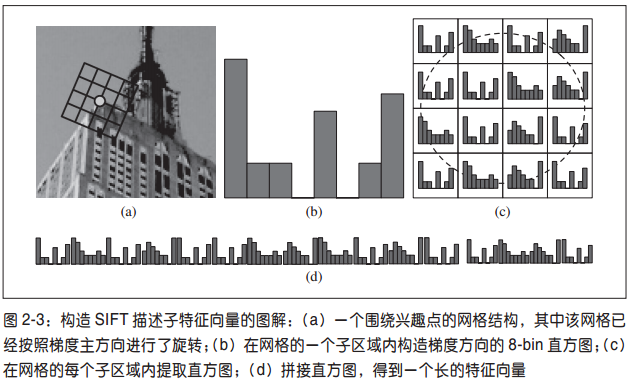### SIFT描述子补充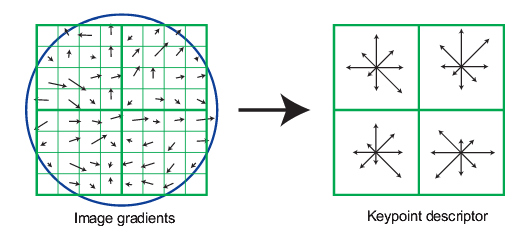## 随机一致性采样（RANdom SAmple Consensus RANSAC）

### RANSAC的基本假设是

• “内群”数据可以通过几组模型的参数来叙述其分布，而“离群”数据则是不适合模型化的数据。
• 数据会受噪声影响，噪声指的是离群，例如从极端的噪声或错误解释有关数据的测量或不正确的假设。
• RANSAC假定，给定一组（通常很小）的内群，存在一个程序，这个程序可以估算最佳解释或最适用于这一数据模型的参数。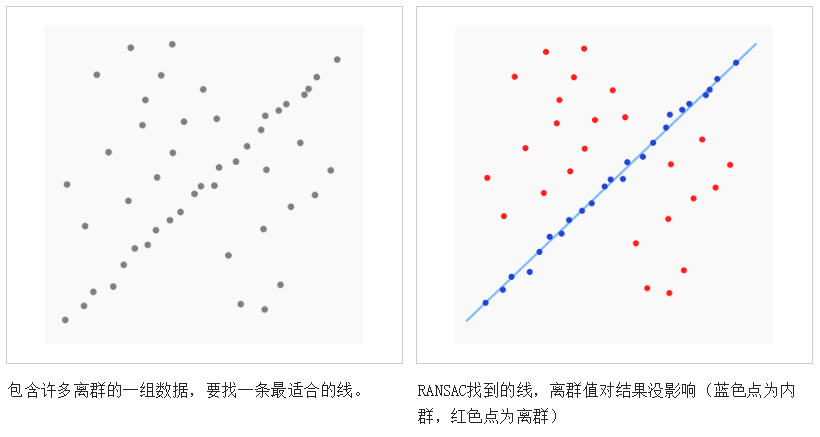### RANSAC优缺点

RANSAC的优点是它能鲁棒的估计模型参数。例如，它能从包含大量局外点的数据集中估计出高精度的参数。RANSAC的缺点是它计算参数的迭代次数没有上限；如果设置迭代次数的上限，得到的结果可能不是最优的结果，甚至可能得到错误的结果。RANSAC只有一定的概率得到可信的模型，概率与迭代次数成正比。RANSAC的另一个缺点是它要求设置跟问题相关的阀值。
RANSAC只能从特定的数据集中估计出一个模型，如果存在两个（或多个）模型，RANSAC不能找到别的模型。

## 最小二乘法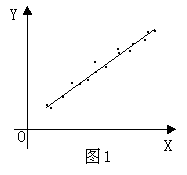$y'=a_0+a_1x$----------------------------------(最1)

$\varphi = \Sigma(y_i-y'_i)^2$-----------------------------(最2)

$\varphi = \Sigma(y_i-a_0-a_1x_i)^2$---------------------(最3)
$\varphi$分别对$a_0,a_1$求偏导，令两个偏导数为0，即：
$\frac{\partial \varphi}{\partial a_0}=-2\Sigma(y_i-a_0-a_1x_i)=0$-----------(最4)
$\frac{\partial \varphi}{\partial a_1}=-2x_i\Sigma(y_i-a_0-a_1x_i)=0$--------(最5)

$na_0+(\Sigma x_i)a_1=\Sigma y_i$
$(\Sigma x_i)a_0+(\Sigma x_i^2)a1=\Sigma(x_i,y_i)$

$a_0=\frac{\Sigma y_i-a_1\Sigma x_i}{n}$
$a_1=\frac{\Sigma {x_iy_i}-\Sigma x_i\Sigma y_i/n}{\Sigma x_i^2-(\Sigma x_i)^2/n}$

### 维基百科上最小二乘法应用在线性回归上的例子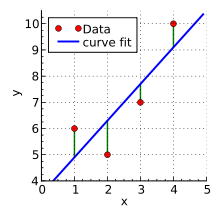$\beta_1+1\beta_2=6$
$\beta_1+2\beta_2=5$
$\beta_1+3\beta_2=7$
$\beta_1+4\beta_2=10$

$S(\beta_1,\beta_2) = [6-(\beta_1+1\beta_2)]^2+[5-(\beta_1+2\beta_2)]^2+[7-(\beta_1+3\beta_2)]^2+[10-(\beta_1+4\beta_2)]^2$

$\frac{\partial S}{\partial \beta_1}=8\beta_1+20\beta_2-56=0$
$\frac{\partial S}{\partial \beta_2}=20\beta_1+60\beta_2-154=0$

$\beta_1=3.5,\beta_2=1.4$• 私有
• 公开
• 删除